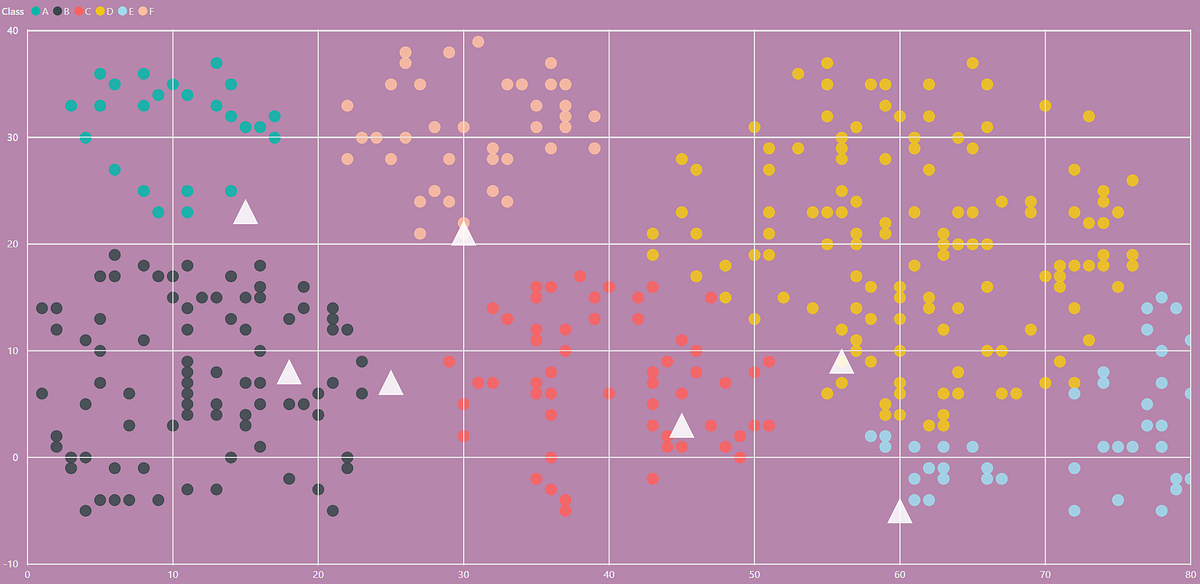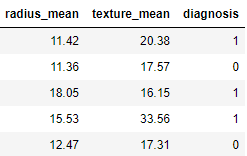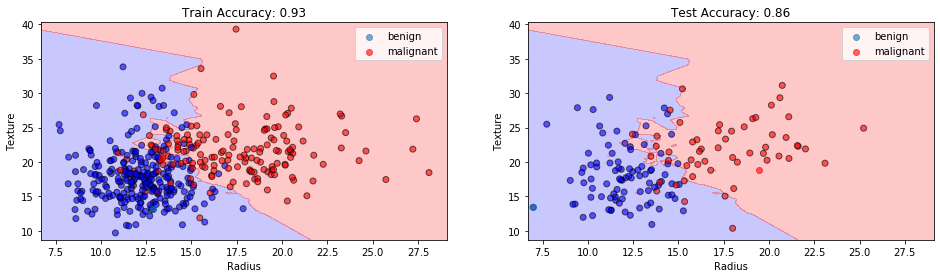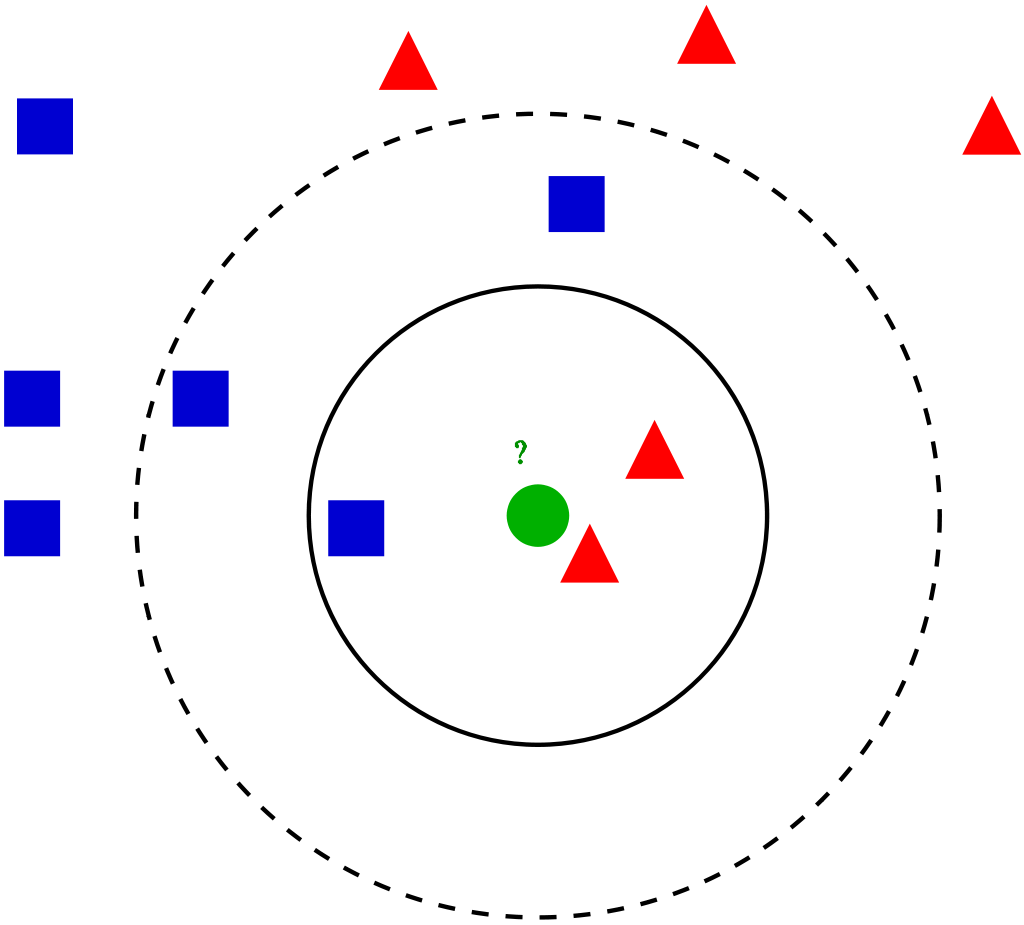1593379860

# Implementing K-Nearest Neighbor Classification Algorithms Using DAX

This article will introduce how to implement KNN(K-nearest neighbor) classification using Data Analysis Expressions (DAX). You can see the color scatter plot in the image below, where each scatters represents each product. The horizontal axis represents the sales quantity, the vertical axis represents the profit, and the remaining 7 white triangles are the test data to be classified. Next, I will use the KNN algorithm to classify these test data.

#power-bi-tutorials #dax #knn-algorithm #power-bi #algorithms

## Buddha Community1593379860

## Implementing K-Nearest Neighbor Classification Algorithms Using DAX

This article will introduce how to implement KNN(K-nearest neighbor) classification using Data Analysis Expressions (DAX). You can see the color scatter plot in the image below, where each scatters represents each product. The horizontal axis represents the sales quantity, the vertical axis represents the profit, and the remaining 7 white triangles are the test data to be classified. Next, I will use the KNN algorithm to classify these test data.

#power-bi-tutorials #dax #knn-algorithm #power-bi #algorithms1602745200

## Exploring The Brute Force K-Nearest Neighbors Algorithm

Did you find any difference between the two graphs?

Both show the accuracy of a classification problem for K values between 1 to 10.

Both of the graphs use the KNN classifier model with ‘Brute-force’ algorithm and ‘Euclidean’ distance metric on same dataset. Then why is there a difference in the accuracy between the two graphs?

Before answering that question, let me just walk you through the KNN algorithm pseudo code.

I hope all are familiar with k-nearest neighbour algorithm. If not, you can read the basics about it at https://www.analyticsvidhya.com/blog/2018/03/introduction-k-neighbours-algorithm-clustering/.

We can implement a KNN model by following the below steps:

2. Initialise the value of k
3. For getting the predicted class, iterate from 1 to total number of training data points
4. Calculate the distance between test data and each row of training data. Here we will use Euclidean distance as our distance metric since it’s the most popular method. Some of the other metrics that can be used are Chebyshev, cosine, etc.
5. Sort the calculated distances in ascending order based on distance values
6. Get top k rows from the sorted array
7. Get the most frequent class of these rows
8. Return the predicted class

#2020 oct tutorials # overviews #algorithms #k-nearest neighbors #machine learning #python1593571140

## K-Nearest Neighbors

A perfect opening line I must say for presenting the K-Nearest Neighbors. Yes, that’s how simple the concept behind KNN is. It just classifies a data point based on its few nearest neighbors. How many neighbors? That is what we decide.

Looks like you already know a lot of there is to know about this simple model. Let’s dive in to have a much closer look.

Before moving on, it’s important to know that KNN can be used for both classification and regression problems. We will first understand how it works for a classification problem, thereby making it easier to visualize regression.

## KNN Classifier

The data we are going to use is the Breast Cancer Wisconsin(Diagnostic) Data Set_. _There are 30 attributes that correspond to the real-valued features computed for a cell nucleus under consideration. A total of 569 such samples are present in this data, out of which 357 are classified as ‘benign’ (harmless) and the rest 212 are classified as _‘malignant’ _(harmful).

The diagnosis column contains ‘M’ or ‘B’ values for malignant and benign cancers respectively. I have changed these values to 1 and 0 respectively, for better analysis.

Also, for the sake of this post, I will only use two attributes from the data → ‘mean radius’ and ‘mean texture’. This will later help us visualize the decision boundaries drawn by KNN. Here’s how the final data looks like (after shuffling):Let’s code the KNN:

``````# Defining X and y
X = data.drop('diagnosis',axis=1)
y = data.diagnosis

# Splitting data into train and test
from sklearn.model_selection import train_test_split
X_train, X_test, y_train, y_test = train_test_split(X,y,test_size=0.25,random_state=42)
# Importing and fitting KNN classifier for k=3
from sklearn.neighbors import KNeighborsClassifier
knn = KNeighborsClassifier(n_neighbors=3)
knn.fit(X_train,y_train)
# Predicting results using Test data set
pred = knn.predict(X_test)
from sklearn.metrics import accuracy_score
accuracy_score(pred,y_test)
``````

The above code should give you the following output with a slight variation.

``````0.8601398601398601
``````

What just happened? When we trained the KNN on training data, it took the following steps for each data sample:

1. Calculate the distance between the data sample and every other sample with the help of a method such as Euclidean.
2. Sort these values of distances in ascending order.
3. Choose the top K values from the sorted distances.
4. Assign the class to the sample based on the most frequent class in the above K values.

Let’s visualize how KNN drew a decision boundary on the train data set and how the same boundary is then used to classify the test data set.KNN Classification at K=3. Image by Sangeet Aggarwal

With the training accuracy of 93% and the test accuracy of 86%, our model might have shown overfitting here. Why so?

When the value of K or the number of neighbors is too low, the model picks only the values that are closest to the data sample, thus forming a very complex decision boundary as shown above. Such a model fails to generalize well on the test data set, thereby showing poor results.

The problem can be solved by tuning the value of _n_neighbors _parameter. As we increase the number of neighbors, the model starts to generalize well, but increasing the value too much would again drop the performance.

Therefore, it’s important to find an optimal value of K, such that the model is able to classify well on the test data set. Let’s observe the train and test accuracies as we increase the number of neighbors.

#knn-algorithm #data-science #knn #nearest-neighbors #machine-learning #algorithms1598035080

## K-Nearest Neighbors Classification From Scratch

This post aims to explore a step-by-step approach to create a K-Nearest Neighbors Algorithm without the help of any third-party library. In practice, this Algorithm should be useful enough for us to classify our data whenever we have already made classifications (in this case, color), which will serve as a starting point to find neighbors.

For this post, we will use a specific dataset which can be downloaded here. It contains 539 two dimensional data points, each with a specific color classification. Our goal will be to separate them into two groups (train and test) and try to guess our test sample colors based on our algorithm recommendation.

## Train and test sample generation

We will create two different sample sets:

• Training Set: This will contain 75% of our working data, selected randomly. This set will be used to generate our model.
• Test Set: Remaining 25% of our working data will be used to test the out-of-sample accuracy of our model. Once our predictions of this 25% are made, we will check the “percentage of correct classifications” by comparing predictions versus real values.
``````## Load Data
RGB\$x <- as.numeric(RGB\$x)
RGB\$y <- as.numeric(RGB\$y)
## Training Dataset
smp_siz = floor(0.75*nrow(RGB))
train_ind = sample(seq_len(nrow(RGB)),size = smp_siz)
train =RGB[train_ind,]
## Testting Dataset
test=RGB[-train_ind,]
OriginalTest <- test
paste("Training and test sets done")
``````

## Training Data

We can observe that our train data is classified into 3 clusters based on colors.

#classification-algorithms #unsupervised-learning #machine-learning #data-science #k-nearest-neighbours #deep learning1597235100

## k nearest neighbors computational complexity

### Understanding the computational cost of kNN algorithm, with case study examplesVisualization of the kNN algorithm (source)

## Algorithm introduction

kNN (k nearest neighbors) is one of the simplest ML algorithms, often taught as one of the first algorithms during introductory courses. It’s relatively simple but quite powerful, although rarely time is spent on understanding its computational complexity and practical issues. It can be used both for classification and regression with the same complexity, so for simplicity, we’ll consider the kNN classifier.

kNN is an associative algorithm — during prediction it searches for the nearest neighbors and takes their majority vote as the class predicted for the sample. Training phase may or may not exist at all, as in general, we have 2 possibilities:

1. Brute force method — calculate distance from new point to every point in training data matrix X, sort distances and take k nearest, then do a majority vote. There is no need for separate training, so we only consider prediction complexity.
2. Using data structure — organize the training points from X into the auxiliary data structure for faster nearest neighbors lookup. This approach uses additional space and time (for creating data structure during training phase) for faster predictions.

We focus on the methods implemented in Scikit-learn, the most popular ML library for Python. It supports brute force, k-d tree and ball tree data structures. These are relatively simple, efficient and perfectly suited for the kNN algorithm. Construction of these trees stems from computational geometry, not from machine learning, and does not concern us that much, so I’ll cover it in less detail, more on the conceptual level. For more details on that, see links at the end of the article.

In all complexities below times of calculating the distance were omitted since they are in most cases negligible compared to the rest of the algorithm. Additionally, we mark:

• `n`: number of points in the training dataset
• `d`: data dimensionality
• `k`: number of neighbors that we consider for voting

## Brute force method

Training time complexity: `O(1)`

**Training space complexity: **`O(1)`

Prediction time complexity: `O(k * n)`

Prediction space complexity: `O(1)`

Training phase technically does not exist, since all computation is done during prediction, so we have `O(1)` for both time and space.

Prediction phase is, as method name suggest, a simple exhaustive search, which in pseudocode is:

``````Loop through all points k times:
1\. Compute the distance between currently classifier sample and
training points, remember the index of the element with the
smallest distance (ignore previously selected points)
2\. Add the class at found index to the counter
Return the class with the most votes as a prediction
``````

This is a nested loop structure, where the outer loop takes `k` steps and the inner loop takes `n` steps. 3rd point is `O(1)` and 4th is `O(## of classes)`, so they are smaller. Therefore, we have `O(n * k)` time complexity.

As for space complexity, we need a small vector to count the votes for each class. It’s almost always very small and is fixed, so we can treat is as a `O(1)` space complexity.

#k-nearest-neighbours #knn-algorithm #knn #machine-learning #algorithms